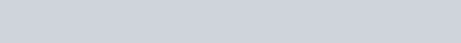With Phase II of the Client Relationship Model (CRM II) fast approaching, Canadian investors will likely be on their own when trying to make sense of their reported rates of return (which will generally be meaningless for benchmarking purposes).  The Modified Dietz rate of return calculator (available in the Calculators section of the blog) continues to be my recommended choice for DIY investors who want to calculate their annual return in a given year.  But once they have a long string of annual returns, how do they go about calculating an average (or “annualized”) return?

Enter the geometric average annual rate of return.  For those investors who still have their G-card, this can be a terrifying equation to tackle.  I’ll admit that the equation has no place in everyday life – it should be restricted to Excel spreadsheets and only allowed to see the light of day once a year (preferably after year-end).

Geometric Average Annual Rate of Return:Where:

r = Annual rate of return in year i

n = Number of years in the measurement period

I find it best to just jump right into an example when trying to understand how to calculate this return.  Let’s assume an investor has calculated the following annual returns over the past 10 years:

 Year Annual return 2004 5.33% 2005 19.64% 2006 19.18% 2007 -10.47% 2008 -31.78% 2009 14.97% 2010 11.47% 2011 -8.53% 2012 22.35% 2013 23.81%

The investor now wants to calculate their 10-year annualized return in order to compare it to a suitable benchmark return.  Here are the steps they would take using Excel:

Step 1:  Enter the calendar year in column A

Step 2:  Enter the corresponding annual returns in column B

Step 3:  Enter an equation in column C that adds 1 to each annual return in column B:

=1+B2

=1+B3

.

.

.

Step 4:  In a different cell, multiply all numbers from column C together (this can be done by using the PRODUCT function in Excel).  Take the result to the power of 1 divided by the number of years in the measurement period (in our example, the number of years is 10).  Subtract 1 from this result.  This sounds confusing, but the equation in our example would simplify to:

=PRODUCT(C2:C11)^(1/10)-1

This would result in a 10-year annualized return of 5.00%.

Example:  10-year annualized return calculation using Excel